# Fully Developed Laminar Flow¶

In this tutorial we will be building a case that involves incompressible laminar flow in a channel with a constant heat flux applied. This case uses air as a working fluid and will be simulated using fully dimensional quantities. A diagram of the case is provided in Fig. 1 and the necessary case parameters are provided in Table 1. Note that round numbers have been selected for the fluid properties and simulation parameters for the sake of simplicity.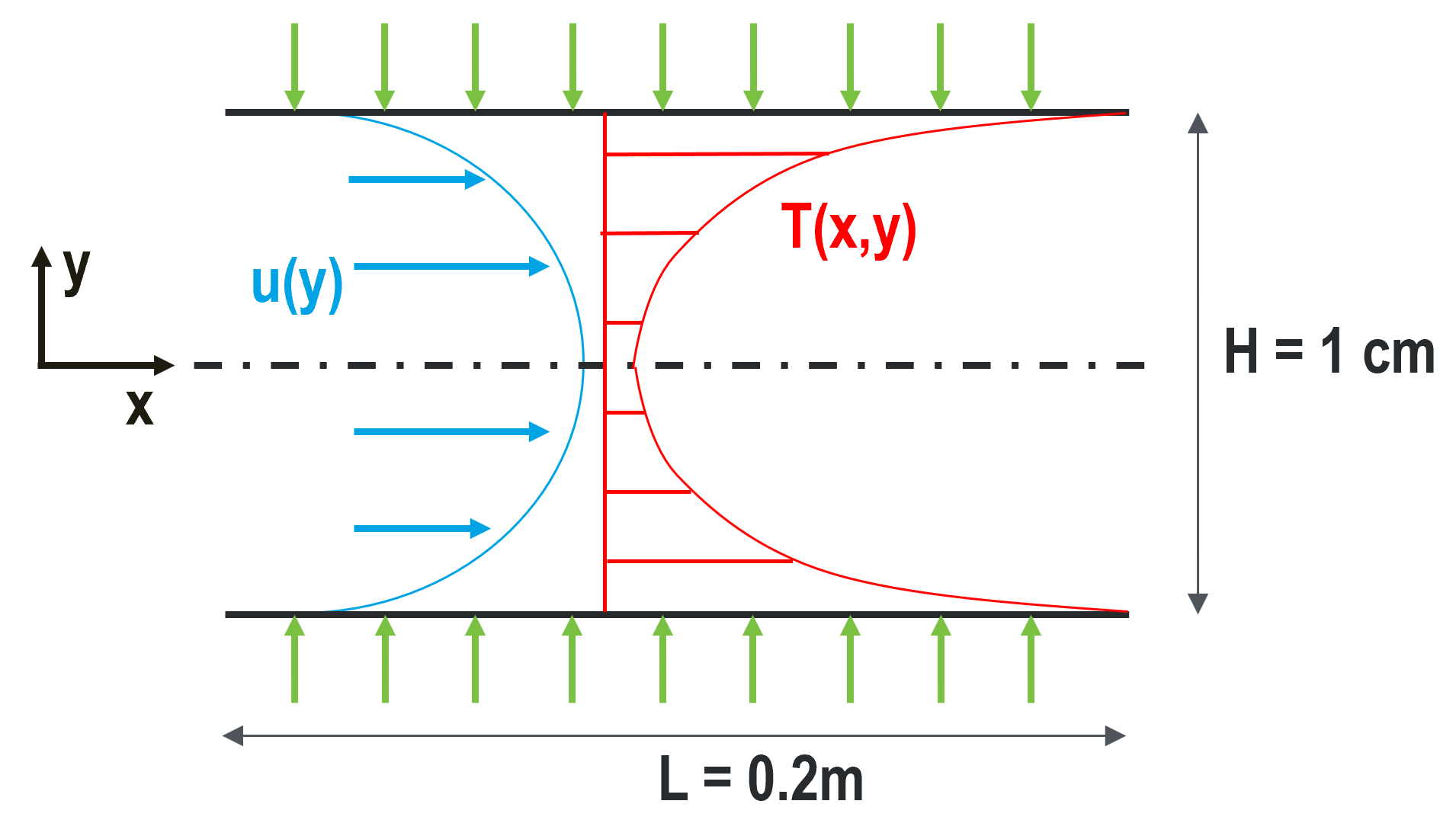Fig. 1 Diagram describing the case setup for fully developed laminar flow in a channel..

Table 1 Fluid properties and simulation parameters
Parameter name variable value
channel height $$H$$ 1 cm
channel length $$L$$ 20 cm
mean velocity $$U_m$$ 0.5 m/s
heat flux $$q''$$ 300 W/m2
inlet temperature $$T_{in}$$ 10 C
density $$\rho$$ 1.2 kg/m3
viscosity $$\mu$$ 0.00002 kg/m-s
thermal conductivity $$\lambda$$ 0.025 W/m-K
specific heat $$c_p$$ 1000 J/kg-K

This case has analytic solutions to the momentum and energy equations which makes it easy to confirm if the problem is setup correctly. These expressions will be used to test the accuracy of the solution.

(1)$u(y) = \frac{3}{2} U_m \left( 1 - 4\left(\frac{y}{H}\right)^2\right)$
(2)$T(x,y)-T_b(x) = \frac{q'' H}{2\lambda}\left( 3\left(\frac{y}{H}\right)^2 - 2\left(\frac{y}{H}\right)^4-\frac{39}{280}\right)$

where the bulk temperature is given by the expression

$T_b(x) = \left(\frac{2q''}{U_m \rho c_p H}\right)x + T_{in}$

## Before You Begin¶

This tutorial assumes that you have installed Nek5000 in your home directory. Be sure that $HOME/Nek5000/bin is in your search PATH. Before running the case, you will need to compile the tools genbox and genmap. These can be compiled using the following command in the $HOME/Nek5000/tools directory:

$./maketools genbox$ ./maketools genmap


Before building the case, the user should set up a case subdirectory in their run directory.

$cd$HOME/Nek5000/run
$mkdir fdlf$ cd fdlf


## Mesh Generation¶

This tutorial uses a simple rectangular box mesh generated by genbox. To create the input file, copy the following script and save the file as fdlf.box.

-2                     spatial dimension (will create box.re2)
2                      number of fields
#
#
#========================================================
#
Box                                       fdlf
-50 -5                                    Nelx  Nely
0.0 0.2 1.0                               x0 x1 ratio
0.0 0.005 0.7                             y0 y1 ratio
v  ,O  ,SYM,W                             Velocity BC's:  (cbx0, cbx1, cby0, cby1)
t  ,O  ,I  ,f                             Temperature BC's:  (cbx0, cbx1, cby0, cby1)


For this mesh we are specifying 50 uniform elements in the stream-wise (x) direction and 5 uniform elements in the span-wise (y) direction. The velocity boundary conditions in the x-direction are a standard Dirichlet velocity boundary condition at xmin and an open boundary condition with zero pressure at xmax. The velocity boundary conditions in the y-direction are a symmetric boundary at ymin and a wall with no slip condition at ymax. The temperature boundary conditions in the x-direction are a standard Dirichlet boundary condition at xmin and an outflow conditon with zero gradient at xmax. The temperature boundary conditions in the y-direction are an insulated condition with zero gradient at ymin and a constant heat flux at ymax. Note that the boundary conditions specified with lower case letters must have values assigned in userbc, which will be shown later in this tutorial. Now we can run genbox with

genbox


When prompted provide the input file name, which for this case is fdlf.box. The tool will produce binary mesh and boundary data file box.re2 which should be renamed to fdlf.re2.

## Control parameters¶

The control parameters for any case are given in the .par file. For this case, create a new file called fdlf.par with the following:

#
# nek parameter file
#
[GENERAL]
dt = 1.0e-4
numsteps = 10000
writeInterval = 2000

userParam01 = 0.01   #channel height [m]
userParam02 = 0.5    #mean velocity [m/s]
userParam03 = 300.0  #heat flux [W/m^2]
userParam04 = 10     #inlet temperature [C]

[VELOCITY]
density = 1.2        #kg/m3
viscosity = 0.00002  #kg/m-s

[TEMPERATURE]
rhoCp = 1200.0       #J/m3-K
conductivity = 0.025 #W/m-K


For this case the properties evaluated are for air at ~20 C. Note that rhoCp is the product of density and specific heat. The userParam list represents an easy way of passing data to Nek5000 that can later be used throughout the .usr file. Additionally, like all values specified in the .par file, they can be changed without the need to recompile Nek5000.

The required values for the initial and boundary conditions specfied by lower case letters in the .box file are defined here as a list of user given parameters, as well as the height of the channel. These initial and boundary conditions will later be called in respective subroutines of the .usr file.

## usr file¶

The user file implements various subroutines to allow the user to interact with the solver. Note that only the subroutines that need to be edited to run this case will be discused. The remaining routines can be left as is.

To get started we copy the template to our case directory

cp $HOME/Nek5000/core/zero.usr fdlf.usr  ## Initial & boundary conditions¶ The next step is to specify the intial conditions. This can be done in the subroutine useric as follows: subroutine useric(ix,iy,iz,ieg) implicit none include 'SIZE' include 'TOTAL' include 'NEKUSE' integer ix,iy,ix,ieg um = uparam(2) Tin = uparam(4) ux = um uy = 0.0 uz = 0.0 temp = Tin return end  The inlet temperature and mean velocity are called from the list of user defined parameters in the .par file. The boundary conditions can be setup in subroutine userbc as follows: subroutine userbc(ix,iy,iz,iside,eg) ! set up boundary conditions implicit none include 'SIZE' include 'TOTAL' include 'NEKUSE' integer ix,iy,iz,iside,eg H = uparam(1) !channel height um = uparam(2) !mean velocity qpp = uparam(3) !heat flux Tin = uparam(4) !mean inlet temperature con = cpfld(2,1) !thermal conductivity term = qpp*H/(2*con) ux = um*3./2.*(1-4.*(y/H)**2) uy = 0.0 uz = 0.0 temp = term*(3.*(y/H)**2-2.*(y/H)**4-39./280.)+Tin flux = qpp return end  The channel height, mean velocity, heat flux, and mean inlet temperature are all called from the list of user defined parameters in the .par file as well. ## SIZE file¶ It is recommended to copy a template of the SIZE file from the core directory and rename it SIZE in the working directory: cp$HOME/Nek5000/core/SIZE.template SIZE


Then, adjust the following parameters in the BASIC section

...

! BASIC
parameter (ldim=2)
parameter (lx1=8)
parameter (lxd=12)
parameter (lx2=lx1-0)

parameter (lelg=250)
parameter (lpmin=1)
parameter (lelt=lelg/lpmin + 3)
parameter (ldimt=1)

...


For this tutorial we have set our polynomial order to be $$N=7$$ which is defined in the SIZE file as lx1=8 which indicates that there are 8 points in each spatial dimension of every element. The number of dimensions is specified using ldim and the number of global elements used is specified using lelg.

## Compilation¶

With the fdlf.usr and SIZE files created, we are now ready to compile:

makenek fdlf


If the compilation is successful, the executable nek5000 will be generated.

## Running the case¶

First, we need to run our domain partitioning tool

genmap


On input specify fdlf as your casename and press enter to use the default tolerance. This step will produce fdlf.ma2 which needs to be generated only once.

Now you can run the case

nekbmpi fdlf 4


To launch an MPI jobs on your local machine using 4 ranks. The output will be redirected to logfile.

## Post-processing the results¶

Once execution is completed your directory should now contain 5 checkpoint files that look like this:

fdlf0.f00001
fdlf0.f00002
...


The preferred mode for data visualization and analysis with Nek5000 is to use Visit/Paraview. One can use the script visnek, to be found in /scripts. It is sufficient to run:

visnek fdlf


to obtain a file named fdlf.nek5000 which can be recognized in Visit/Paraview. In the viewing window one can visualize the flow-field as depicted in Fig. 2 as well as the temperature profile as depicted in Fig. 3 below.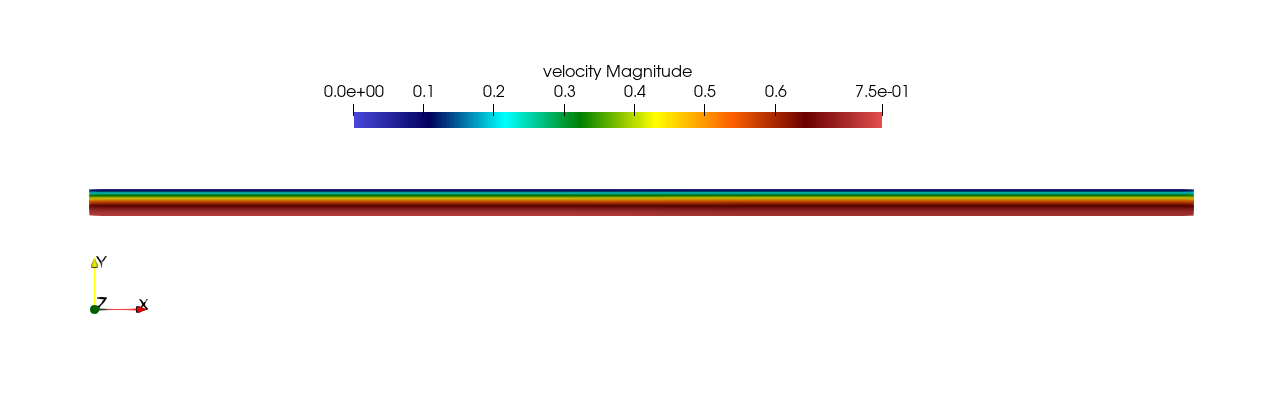Fig. 2 Steady-State flow field visualized in Visit/Paraview. Colors represent velocity magnitude.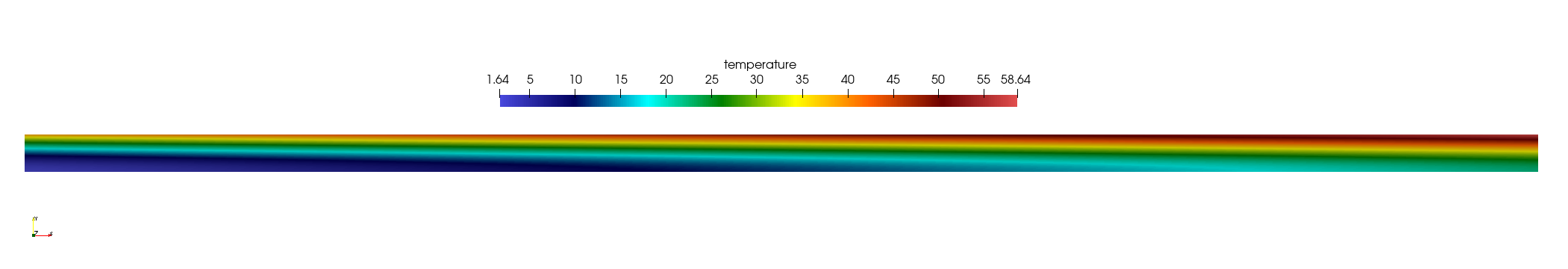Fig. 3 Temperature profile visualized in Visit/Paraview.

Plots of the velocity and temperature varying along the y-axis as evaluated by Nek5000 compared to the analytic solutions provided by Eqs. (1) and (2) respectively are shown below in Fig. 4 and Fig. 5.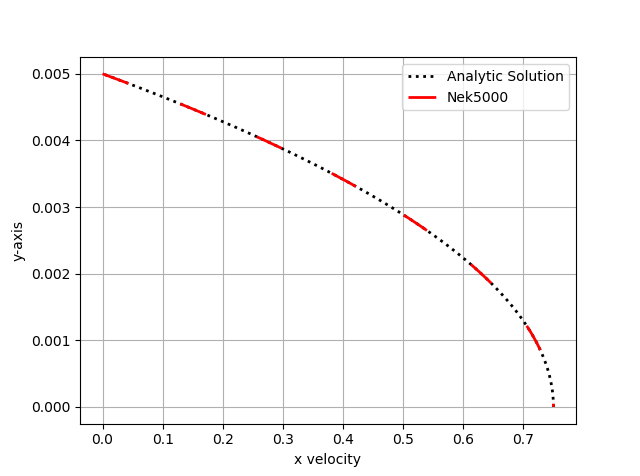Fig. 4 Nek5000 velocity solutions plotted against analytical solutions.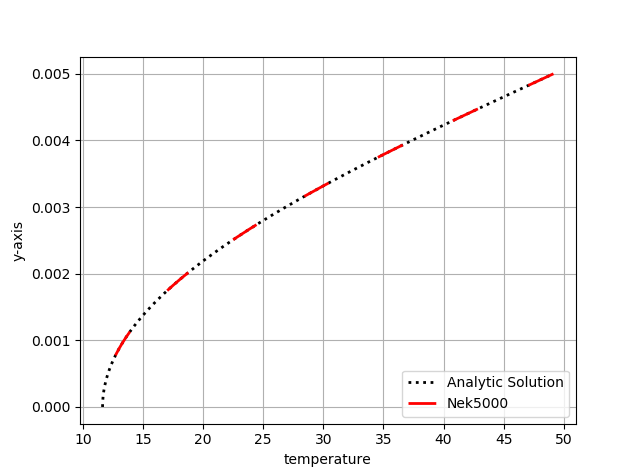Fig. 5 Nek5000 temperature solutions plotted against analytical solutions.